# OEImageTable¶

class OEImageTable


This class represents OEImageTable which is a layout manager that allows to arrange information in rows and columns with variable height and width, respectively.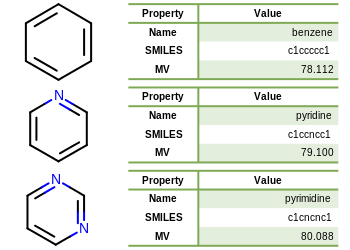Example of using image table

## Constructors¶

OEImageTable(OEImageBase &parent, const OEImageTableOptions &opts)


Creates an OEImageTable object with the specified options.

parent

The OEImageBase object on which the OEImageTable object is placed.

opts

The OEImageTableOptions object that defines the properties and style of the table.

## DrawText¶

bool DrawText(OEImageBase* cell, const std::string& text, double padding = 5.0)


Draws a text to the given table cell. Returns false if the given image does not belong to the table.

Defines the padding used on the left and right of the given cell as a percentage of the width of the cell.

Note

The font that is used to render the text is defined in the OEImageTableOptions class.

## GetBodyCell¶

OEImageBase *GetBodyCell(unsigned int row, unsigned int col)


Returns the OEImageBase for the cell positioned in the $$r^{th}$$ row and $$c^{th}$$ columns of the ‘body’ of the table. A cell is considered to be part of the ‘body’ of the table if it is neither in the header nor in the stub column.

row

This value has to be in the range from $$1$$ to OEImageTable.NumRows(true).

col

This value has to be in the range from $$1$$ to OEImageTable.NumColumns(true).

Note

A null pointer is returned if the row or the col parameters are outside the acceptable range.

Example

The image below marks the cell returned by OEImageTable.GetBodyCell method for row = 1 and col = 2 parameters.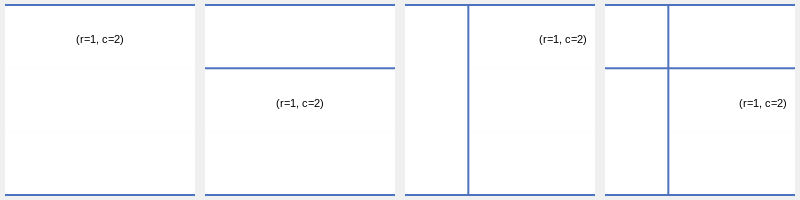Example of accessing a specific ‘body’ cell of the table.

## GetBodyCells¶

OESystem::OEIterBase<OEImageBase> *GetBodyCells(unsigned int row=0,
unsigned int col=0)


Returns an iterator over the ‘body’ cells of the OEImageTable object. A cell is considered to be part of the ‘body’ of the table if it is neither in the header nor in the stub column. The cells are returned from left to right and top to bottom order.

row

This value has to be in the range from $$0$$ to OEImageTable.NumRows(true).

col

This value has to be in the range from $$0$$ to OEImageTable.NumColumns(true).

Note

An empty iterator is returned if the row or the col parameters are outside the acceptable range.

Examples

If the OEImageTable.GetBodyCells method is invoked without any parameter, then all ‘body cells’ are returned.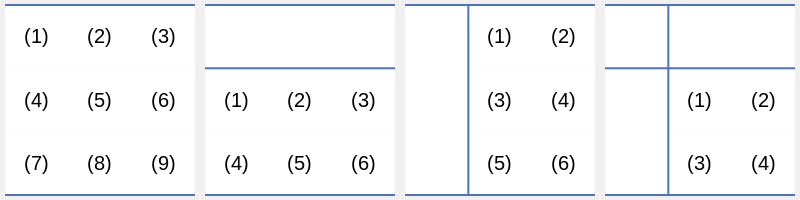Example of accessing all ‘body’ cells of the table

(The numbers inside the cells represent the order in which the cells are returned)

If the row parameter is zero, then the OEImageTable.GetBodyCells method returns an iterator over the ‘body’ cells of the specified column. The image below marks the cells returned by OEImageTable.GetBodyCell method for row = 0 and col = 2 parameters.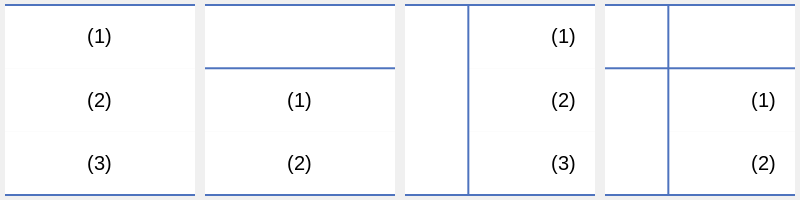Example of accessing ‘body’ cells of a specific columns in the table

(The numbers inside the cells represent the order in which the cells are returned)

If the column parameter is zero, then the OEImageTable.GetBodyCells method returns an iterator over the ‘body’ cells of the specified column. The image below marks the cells returned by OEImageTable.GetBodyCell method for row = 2 and col = 0 parameters.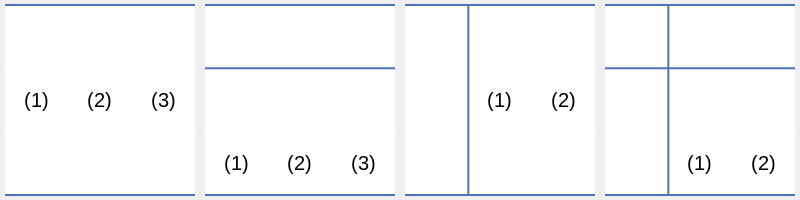Example of accessing ‘body’ cells of a specific row in the table

(The numbers inside the cells represent the order in which the cells are returned)

## GetCell¶

OEImageBase *GetCell(unsigned int row, unsigned int col)


Returns the OEImageBase pointer for the cell positioned in the $$r^{th}$$ row and $$c^{th}$$ columns.

row

This value has to be in the range from $$1$$ to OEImageTable.NumRows().

col

This value has to be in the range from $$1$$ to OEImageTable.NumColumns().

Note

A null pointer is returned if the row or the col parameters are outside the acceptable range.

Example

The image below marks the cell returned by OEImageTable.GetCell method for row = 1 and col = 2 parameters.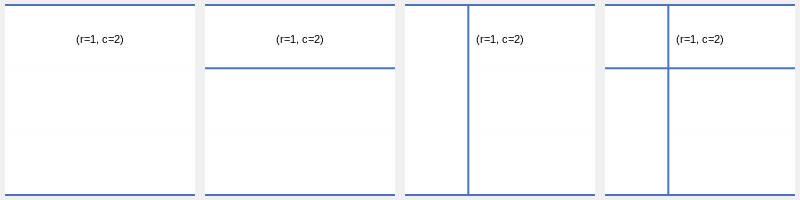Example of accessing a specific cell of the table

## GetCells¶

OESystem::OEIterBase<OEImageBase> *GetCells(unsigned int row=0,
unsigned int col=0)


Returns an iterator over the cells of the OEImageTable object. The cells are returned from left to right and top to bottom order.

row

This value has to be in the range from $$0$$ to OEImageTable.NumRows().

col

This value has to be in the range from $$0$$ to OEImageTable.NumColumns().

Note

An empty iterator is returned if the row or the col parameters are outside the acceptable range.

Examples

If the OEImageTable.GetCells method is invoked without any parameter, then all cells of the table are returned.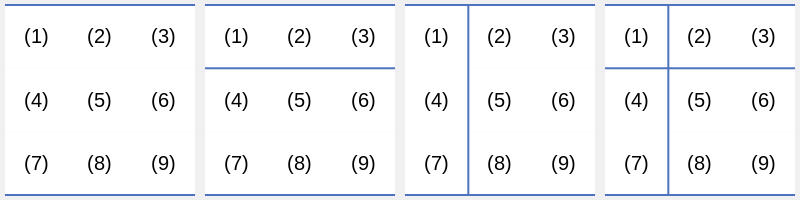Example of accessing all cells of the table

(The numbers inside the cells represent the order in which the cells are returned)

If the row parameter is zero, then the OEImageTable.GetCells method returns an iterator over the cells of the specified column. The image below marks the cells returned by OEImageTable.GetCell method for row = 0 and col = 2 parameters.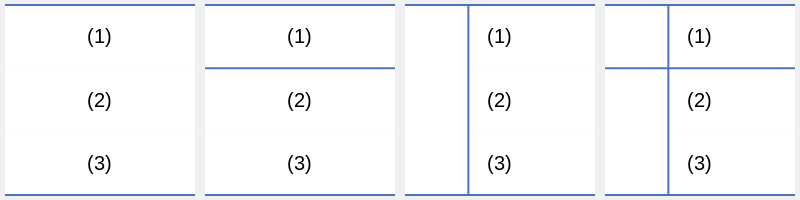Example of accessing all cells of a specific columns of the table.

(The numbers inside the cells represent the order in which the cells are returned)

If the column parameter is zero, then the OEImageTable.GetCells method returns an iterator over the cells of the specified row. The image below marks the cells returned by OEImageTable.GetCell method for row = 2 and col = 0 parameters.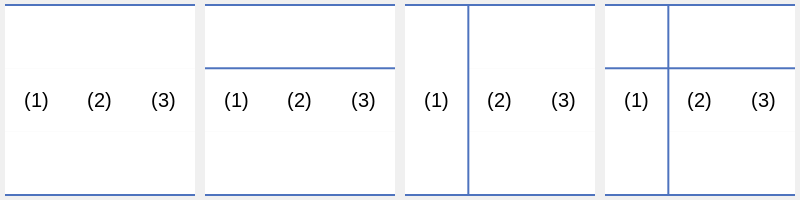Example of accessing all cells of a specific row of the table.

(The numbers inside the cells represent the order in which the cells are returned)

OEImageBase *GetHeaderCell(unsigned int col, bool skipstub=true)


Returns the OEImageBase for the cell positioned in the $$c^{th}$$ columns of the ‘header’ of the table.

col

This value has to be in the range:

skipstub

The parameter that determines whether to consider the stub column. If skipstub is false and the table has a stub column, than that stub column is considered as the first column.

Note

A null pointer is returned if the table has no header or if the col parameters is outside the acceptable range.

Example

The image below marks the cell returned by OEImageTable.GetHeaderCell method for col = 2 and skipstub = true parameters.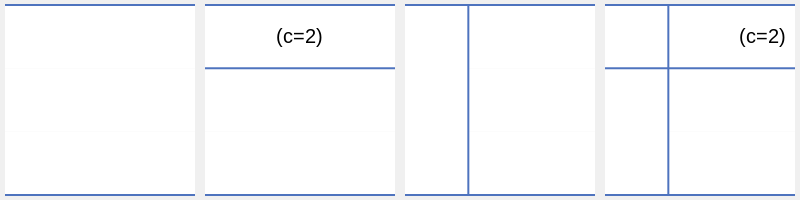Example of accessing a specific header cell of the table

OESystem::OEIterBase<OEImageBase> *GetHeaderCells(bool skipstub=true)


Returns an iterator over the header cells of the OEImageTable object. The cells are returned from left to right order.

skipstub

The parameter that determines whether to consider the stub column. If skipstub is false and the table has a stub column, than that stub column is considered as the first column.

Note

An empty iterator is returned if table has no header row.

Example

The image below marks the cells returned by OEImageTable.GetHeaderCells for skipstub = true parameter.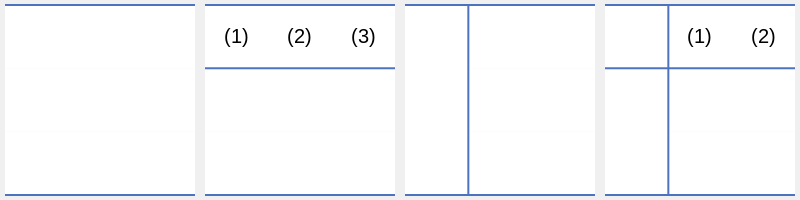Example of accessing header cells of the table

(The numbers inside the cells represent the order in which the cells are returned)

## GetOptions¶

const OEImageTableOptions &GetOptions() const


Returns the options used to initialized the OEImageTable object.

## GetStubColumnCell¶

OEImageBase *GetStubColumnCell(unsigned int row)


Returns the OEImageBase for the cell positioned in the $$r^{th}$$ row of the ‘stub column’ of the table.

row

This value has to be in the range from $$1$$ to OEImageTable.NumRows(true).

Note

A null pointer is returned if the table has no stub column or if the row parameters is outside the acceptable range.

Example

The image below marks the cell returned by OEImageTable.GetStubColumnCell method for row=1 parameter.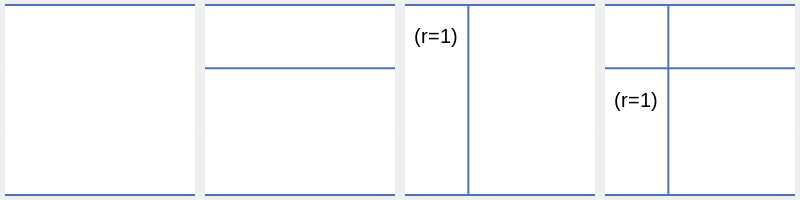Example of accessing a specific stub column cell of the table

## GetStubColumnCells¶

OESystem::OEIterBase<OEImageBase> *GetStubColumnCells()


Returns an iterator over the stub column cells of the OEImageTable object. The cells are returned from top to bottom order.

Note

An empty iterator is returned if table has no stub column.

Example

The image below marks the cells returned by OEImageTable.GetStubColumnCells method.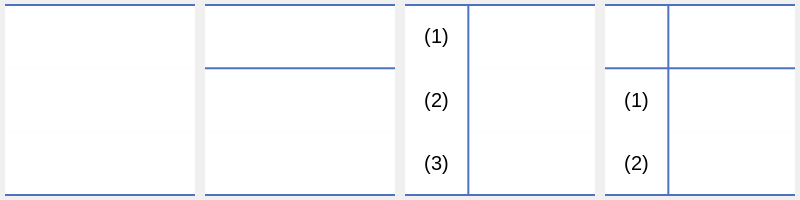Example of accessing stub column cells of the table

(The numbers inside the cells represent the order in which the cells are returned)

## NumColumns¶

unsigned int NumColumns(bool onlybody=false) const


Returns the number of columns of the OEImageTable object.

onlybody

If this parameter is ‘true’, than stub column is not counted if exists.

## NumRows¶

unsigned int NumRows(bool onlybody=false) const


Returns the number of rows of the OEImageTable object.

onlybody

If this parameter is ‘true’, than header row is not counted if exists.